Science Journal of Applied Mathematics and Statistics
Volume 3, Issue 4, August 2015, Pages: 188-193

On One 3-Dimensional Boundary-Value Problem with Inclined Derivatives

Mekhtiyev Magomed Farman, Aliyev Nihan Alipanah, Fomina Nina Ilyinichna

Faculty of Applied Mathematics and Cybernetics, Baku State University, Baku, Azerbaijan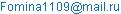(Fomina N. I.)

Mekhtiyev Magomed Farman, Aliyev Nihan Alipanah, Fomina Nina Ilyinichna. On One 3-Dimensional Boundary-Value Problem with Inclined Derivatives. Science Journal of Applied Mathematics and Statistics. Vol. 3, No. 4, 2015, pp. 188-193. doi: 10.11648/j.sjams.20150304.14

Abstract: A boundary-value problem with inclined derivatives in 3-dimensional space with the boundaries – surfaces of Liapunov type is considered in the paper. The method of investigation is based on the necessary conditions. The advantage compared to the theory of potentials is that we don‘t have limit passage, we use boundary values which are obtained from the principal relationships called necessary conditions. Remark that the directions of the derivatives given in the boundary conditions are arbitrary. Tangent directions may be some subset of the given directions.

Keywords: Inclined Derivatives, Normal Derivative, Necessary Conditions, Theory of Potentials, Fredholm Integral Equations of Second Kind

Contents

1. Introduction

As it is known from , , , , , formulas of jumps obtained both for normal derivative of the simple layer potential and for the potential of double layer itself enable to reduce Neumann and Dirichlet boundary-value problems to Fredholm integral equations of second kind. What concerns the problems with inclined derivatives then they were completely investigated only in 2-dimensional case clue to the methods of the theory of functions of complex variable , .

For 3-dimensional case as it was remarked by Bits adze A.B. in  "as there isn’t theory of 3-dimensional analogue of Riemann-Hilbert problem, such reduction is not very useful". This case for a general second order linear differential equation of elliptic type when the given directions on a smooth boundary of the domain makes an acute angle with the outer normals was investigated in , . By means of the concept of a symbol J. Jiro’s results ,  were obtained more simple in , .

The case of a plane system of second order equations when the principal part is Laplace operator was considered in , , . There was considered finiteness of the problem index, explicitly written out the necessary and sufficient condition of the problem solvability and, finally, shown that the problem character is essentially influenced by the presence of minor terms entering the boundary condition if the coefficients at the derivative satisfy some equalities.

Further was determined that if the inclined line is tangent at some finite number of points of boundary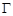or in some finite number of lines onthe given inclination (direction) is tangent for this line then the kernel of the considered operator is finite dimensional, i.e. the solution of the problem is not unique , , . These results of 2-dimensional problems (the start of investigations is in the monographs ) are generalized in , .

In 3-dimensional case the index formula for the problem with inclined derivatives of the system of Laplace equations was given without proof by A.I. Volpert . Further remark that the work of a group of mathematicians  is devoted to the indices of boundary-value problems in abstract case.

The problem with inclined derivative in 3-dimensional Euclid space was investigated by A. Yanuschauskas . There was obtained a condition of non-conditional solvability of the problem. Remark that in  there is given an additional condition along the line lying on the boundary for one-valued solvability and the problem is reduced to a Dirichlet problem.

At last remark that the existing, for instance in , limit theorems for tangent derivative of the potential of a simple and double layer, as well as for the normal derivative of the double layer potential, unfortunately, didn’t find their application for the solution of boundary-value problems for elliptic equations.

The present paper is devoted to the question of solvability of boundary-value problem with inclined derivatives in 3-dimensional space.

The problem statement belongs to Bitsadze A.V. , .

2. Problem Statement and Necessary Conditions

So, consider the following problem: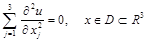(1)(2)

where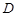is a bounded domain with boundary.

Let there hold true the following restrictions:

I0. The boundaryof the bounded domain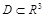is Liapunov surface.

20. The coefficients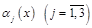of boundary condition (2) belong to some Holder class and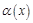is a continuous function.

Then holds true

Theorem 1. On condition I0 any solution to equation (1) defined insatisfies the relationships: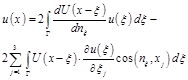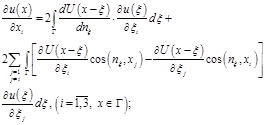(3)

where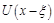is a fundamental solution to equation (1) and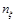is an outer normal to the boundaryat point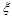.

Really, the first of expressions (3) is known from the general course of equations of Math Physics . For the proof of the second expression let us do the following. Supposing that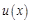is the solution of equation (1) and multiplying both parts of these equations written at pointby function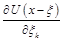whereis a fundamental solution of Laplace equation, integrating by domainand applying Gauss-Ostrogradsky’s formula  we will obtain: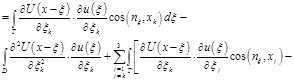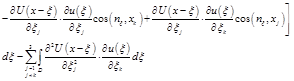or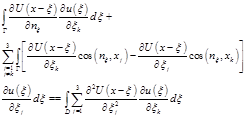From this follows the second expression from (3) if to take in to account thatis fundamental solution of Laplace equation , , .

Thus, for unknown functions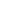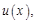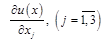we have five relationships: boundary condition (2) and four expressions entering (3). Remark that the last three relationships contain singular integrals.

3. Separation of Singularities

Let us try to replace these 3 relationships with two ones where singular integrals don’t enter. For this, taking in to account that fundamental solution to Laplace equation has the form,

accepting the designations(4)

let us make the following expressions: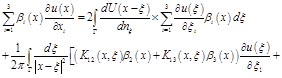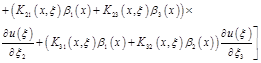(5)

where the unknowns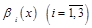are defined from the system: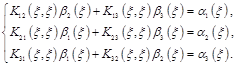(6)

Then from representation (4) there easily follows the properly: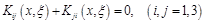,

by means of which the principal determinant of system (6) vanishes, i.e. the rank of the principal matrix is equal to two. For calculation of the rank of the augmented matrix let us designate the unit vectors by directions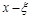andas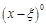and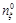respectively.

Then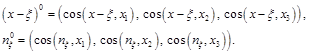Consider the unit vector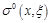defined as follows: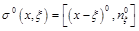.

Taking into account (4), forwe shall obtain: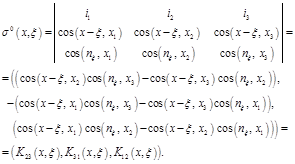Thus,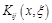are direction cosines of the unit vector lying on the tangent plane, drawn to the boundaryat point. Furthermore, accepting designations:it is easy that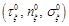form a right triple, i.e. they are orthonormed and directed so that their mixed product is equal to unit.

Now, let us calculate the rank of the augmented matrix of system (6):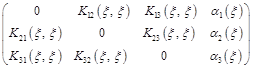.

As the first determinant of third order vanishes let us consider the following determinants: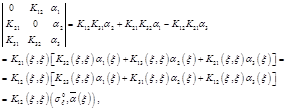where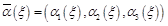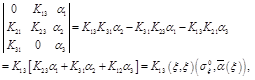and, at last,From the obtained expressions it follow that for compatibility of system (6) by Croneker-Capelli theorem the rank of the augmented matrix must be equal to two.

Then, taking in to account that vectoris unit, we obtain: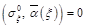(7)

4. Regularization

As it is seen from the problem statement, vector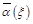doesn’t depend on us. So, relationship (7) to hold true it is sufficient for each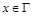to tend point(is the integral variable) to point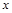by reasonable way.

Then from system (6) (this system in vector designations has the form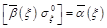for the vector)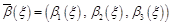we obtain two linear-independent solutions.

Let us designate them as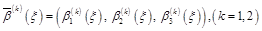(8)

Remark that if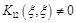then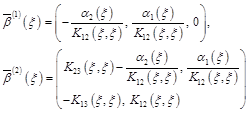are linear-independent solutions of system (6).

Asis a unit vector then one of the component must be different from zero.

Thus, taking into account (8) from (5) we obtain: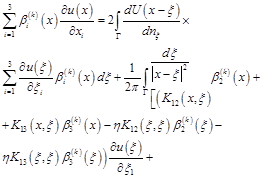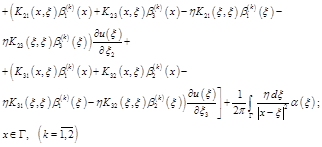where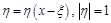.

In this way there is proved

Theorem 2. On conditions 10– 20 for the solution of problem (1) – (2) there hold true the following regular relationships: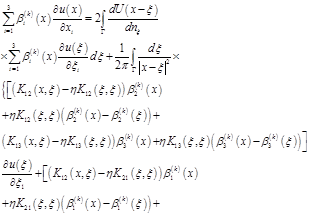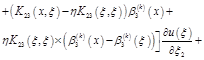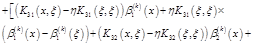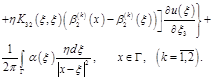(9)

Theorem 3. On conditions 10– 20 if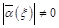then vectors,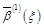and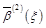are linear independant.

Indeed, without loss of generality, supposing that, we have: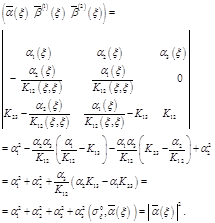Remark: The statement of theorem 3 is equivalent to Lopatinsky’s condition .

Remark: In this way there are given sufficient conditions for the validity of system (7.73) from Bitsadze A.V. book  and defined the coefficients of this system.

5. Fredholm Property

Theorem 4. On the conditions of theorem 3 ifbelongs to some Holder class then the stated problem (1) – (2) has Fredholm property.

Indeed, it follows from theorem 3 that relationships (2) and (9) enable to obtain a normal system of Fredholm integral equations of second kind for functions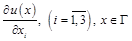. If to adjoin the first expression from (3) to the obtained a compact, i.e. complete system. The obtained system is a Fredholm one of second kind and the kernel contains a weak singularity.

Remark: If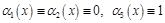then, evidently, from (7),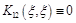, and for validity of system (6)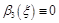. In this case system (6) has the unique normal solution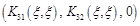. That is why the statement of theorem 3 doesn’t hold true and the stated problem is not Fredholm.

Theorem 5. If on conditions 10– 20 the obtained system of Fredholm integral equations of second kind is solvable then the solution of boundary-value problem (1) – (2) is represented in the form: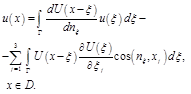(10)

Remark: If the statement of theorem 3 doesn’t hold true along some manifold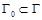then an additional boundary condition onshould be given for Fredholm property of the stated problems.

By this method there were investigated various problems some of which we enumerate:

1. Boundary-value problems with non-local conditions for an equation of elliptic type , , .

2. Boundary-value problems for integro-differential equation with partial derivatives with nonlocal and global addends in a boundary condition , .

3. Cauchy problem and boundary-value problem for Navie-Stoks equation , , , , .

4. Cauchy problem and mixed problem for an equation of hyperbolic type , , .

5. Stephan’s inverse problem , .

References

1. Giraud G. Equations a integrales, Ann, Suent.Ecale narm, Super, 51, et 4, fase 3,1934, 251-372.
2. Bitsadze A.V. Boundary value problems for second elliptic equations. North-Holland, 1968.
3. Vladimirov V.S. Equations of Mathematical Physics. Mir Publisher, Moscow, 1984.
4. Bitsadze A.V. Equations of math.physics. Nauka, Moscow, 1976, 280 p.
5. Bitsadze A.V. Some classes of partial Differential Equations. Moscow, 1981,448 p.
6. Giraud G. Sur certains operations du tupe elliptigul, C.r.Acad, Sci, 200,1935, 1651-1653.
7. Mikhlin S.G. To the theory of multidimensional singular integral equations. News of Leningrad State Univ., №1, 1956, p.3-24.
8. Mikhlin S.G. Multidimensional singular integrals and integral equations. Pergamon, 1965.
9. Bitsadze A.V. Boundary value problems for systems of linear differential equations of elliptic type. News of the Academy of Sciences of Georgian SSR, 5, 8, 1944, p.761-770.
10. Verkua N.P. Systems of singular integral equations. M.-L ., Gostechizdat, 1950, p.396 .
11. Yegorov Yu.V., Kondratiev V.A. A problem with angled derivative, Math.book, 1969, vol.78, p.148- 179.
12. Bitsadze A.V. To the theory of non Fredholm elliptic boundary value problems.Dif. Eq-s with partial derivatives, Proceedings of symposium dedicated to the 60-th anniversary of acad.S.L.Sobolev, Nauka, Moscow, 1976, p.69-70.
13. Verkua I.N. New methods for solution of elliptic equations. North-Holland, Amsterdam, 1967.
14. Tovmasian N.Y. General boundary value problem for elliptic systems of second order with constant coefficients. Dif.eq., vol.2, 1966, №1, 3-23, №2, 163-171.
15. Volpert A.I. On the index of boundary value problems for systems of harmonic functions with three independent variables. DAN SSSR, 133, №1, p.13-15.
16. Paleh R. Seminar on Atie-Zinger theorem on index. Mir, Moscow, 1970, 362 p.
17. Yanushauskas A.On unconditional solvability of problems with inclined derivative. Dif. eq., vol.3, 1967, №1, p. 86-96.
18. Courant R. Rartial differential equations. New York - London, 1962.
19. Bitsadze A.V. To a problem with inclined derivative for harmonic functions in three- dimensional domains. Materials of joint Soviet-American symposium on equations in partial derivatives. Siber.depart.of SSSR Academy of Science, Novosibirsk, 1963, p.133-136.
20. Lopatinsky Ya.B. On one method of reducing boundary value problems for systems of elliptic type equations to regular integral equations. Ukr.Math.journ., 1953, vol.5,p.123-151.
21. Aliyev N., Jahanshahi M. Solution of Poisson’s equation with global, local and nonlocal boundary conditions // International Journal of Mathematical Education in Science and Technology, 33 (2002), no.2, p.241-247.
22. Jahanshahi M., Aliyev N. Determining of an analytic function on its analytic do main by Cauchy-Riemann equation with special kind of boundary conditions // Southeast Asian Bulletin Mathematics,28 (2004), no.1, p.33-39.
23. Hosseini S.M., Aliyev N. Sufficient conditions for the reduction of a BVP for PDE with non-local and global boundary conditions to Fredholm integral equations (on a rectangular domain). Applied Mathematics and Computation //147 (2004), no.3, p.669-685.
24. Magomed F. Mekhtiyev, Nihan A.Aliyev, Mehran R.Fatemi. On Fredholm property of a Boundary Value Problem for a first order equation with general boundary conditions // Transactions of National Academy of Sciences of Azerbaijan, 2009, Baku, vol.XXIX, no.4, p.221-226.
25. M.R.Fatemi and N.A.Aliyev. General Linear Boundary Value Program for the Second-Order Integro-Differential Loaded Equation with Boundary Conditions // Containing Both Nonlocal and Global Terms // Hindawi publishing Corporation. Abstract and Applied Analysis, 2010, 12 pages.
26. Aliyev N., Hosseini S.M. Cauchy problem for the Navier-Stokes equation and its reduction to a non- linear system of second kind Fredholm integral equations // International Journal of Pure and Applied Mathematics 3 (2002), no.3, p.317-324.
27. Aliev N., Rezapour Sh., Jahanshahi M. On Fefferman’s Non-existence Problems. Mathematica Moravica Journal of University of Kragujevac, Serbia, Vol. 11 (2007), p.1-7.
28. Aliev N., Rezapour Sh., Jahanshahi M. A Mixed Problem for Navier-Stokes system // Mathematica Moravica Journal of University of Kragujevac, Serbia, Vol. 12-2 (2008), p. 1-14.
29. Aliev N., Rezapour Sh., Jahanshahi M. On a mixed problem for Navier-Stokes System in the Unit Cube // Mathematica Moravica, Vol. 13-1 (2009), 13-24.
30. M. Jahanshahi, N.Aliev. An Analytic Method for Investigation and Solving Two-Dimensional Steady State Navier-Stokes Equations I // Southeast Asian Bulletin of Mathematics (2009) 33: p.749-763 (1075-1089).
31. Aliyev N., Hosseini S.M. An analysis of a parabolic problem with a general (non-local and global) supplementary linear conditions I // Italian Journal of Pure and Applied Mathematics No. 12 (2002), p.143-153 (2003)
32. Aliyev, N., Hosseini S.M. An analysis of a parabolic problem with a general (non-local and global) supplementary linear conditions II // Italian Journal of Pure and Applied Mathematics No.13 (2003), p.115-127.
33. Bahrami F., Aliyev N., Hosseini S.M. A method for the reduction of four dimensional mixed problems with general boundary conditions to a system of second kind Fredholm integral equations // Italian Journal of Pure and Applied Mathematics No.17 (January 2005), p. 91-104.
34. K.Ivaz, N.Aliyev. Nonlinear Two-Phase Stefan Problem // Journal of Sciences, Islamic Republic of Iran; Autumn 2007, Vol.18, No. 4, p.329-337.
35. K.Ivaz, N. Aliyev. Control of the Moving Boundary // Southeast Asian Bulletin of Mathematics (2010) 34: p. 439-450.

 Contents 1. 2. 3. 4. 5.
Article ToolsAbstractPDF(197K)